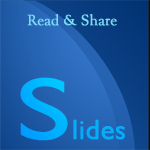# Go Language, An Introduction

This presentation is an introduction on GO language, covering variable declarations, built-in types, type conversions, functions, functions and closures, packages, control structures, maps, structs, interfaces, Error Handling etc.

## Transcript

Go Language, An Introduction

Hello World

File : hello.go

```package main
import "fmt"
func main(){
fmt.Println("Hello Go!!")
}```

Output : Hello Go!!

Variable Declaration

Variable

Variable declaration and initialization

```// Declare types after identifier
var foo int = 52 // declare the variable with initialization
var foo int // declare the variable without initialization
var foo, bar int = 56, 9999 // declare more than one variable
var foo = 72 // type omitted, will be inferred
foo := 72 // shorthand, only in func bodies
const constant = "Declare constant this way"```

Built-in Types

```int int8 int16 int32 int64
uint uint8 uint16 uint32 uint64 uintptr
float32 float64
complex64 complex128
bool
string
byte // alias for uint8
rune // alias for int32 ~= a character (Unicode code point) - very Viking
```

Type Conversions

```var x int = 72
var y float64 = float64(x)
var z uint = uint(y)
// You can use the following syntax also (alternative syntax)
x := 72
y := float64(x)
z := uint(y)
```

Functions

Declaring a simple function

```func MyfunctionName() {}
```

Function with parameters

Function with multiple parameters (different types)

```func MyfunctionName(parameter1 int, parameter2 string) {}
```

Function with multiple parameters (same type)

```func MyfunctionName(parameter1, parameter2 string) {}
```

Function and value return

Function with return type declaration

```func MyfunctionName() (string) {
return "return string"
}
```

Function with multiple return values

```func MyfunctionName() (int, string) {
return 200, "Pizza"
}
var a, str1 = MyfunctionName()
```

Return multiple results, by return

```func MyfunctionName() (x int, str1 string) {
x = 200
str1 = "Pizza"
// x and str1 will be returned
return
}
var x, str1 = MyfunctionName()
```

Functions As Values And Closures

Functions As Values

Call a function :

```func main() {
// assign a function to a name
add := func(x, y int) int {
return x + y
}
//call the function
}
```

Functions as Values and Closures

Go supports anonymous functions, which can form closures. Anonymous functions are useful when you want to define a function inline without having to name it.

```func add() func(int) int {
sum := 0
return func(a int) int {
sum += a // sum is declared outside, but still visible
return sum
}
}
```

Packages

```● Declare package at top of every source file
● executables are in package main
● convention: package name == last name of import path
● upper case identifier: exported (visible from other packages)
● Lower case identifier: private (not visible from other packages)
```

Control structures

if statement

Simple if statement

```if x > 0 {
return x
} else {
return -x
}
```

It is possible to put one statement before the condition

```if x := y + z; z < 100 {
return a
} else {
return z - 100
}
```

for Loops

Only `for` loop is available, no `while`, no `until`

```for x := 1; x < 10; x++ {
}
for ; x < 10; { // while - loop
}
for x < 10 { // omit semicolons if there is only a condition
}
for { // you can omit the condition ~ while (true)
}
```

Switch statement

```switch gradeSystem{
case "A":
// cases break automatically
case "B":
default:
}
```

Arrays

```var x int // declare an int array of lenght 10.
x = 100 // set elements
i := x // read elements
// declare and initialize
x := int{1, 2}
x := [...]int{1, 2} // elipsis -> Compiler figures out array length
```

Maps

```var m map[string]int
m = make(map[string]int)
m["key"] = 200
fmt.Println(m["key"])
delete(m, "key")
elem, ok = m["key"] // retrive the 'key' if it is present
// map literal
var m = map[string]Vertex{
"Singapur": {1.3000, 103.8000},
"Slovakia": {45.1500, 17.1167},
}
```

Structs

There are no classes, only structs. Declare, create and access.

```// Declaration
type point struct {
X, Y int
}
// Creating
var h = point{1, 2}
// Accessing members
h.X = 4
```

Methods on structs

There are no classes, only structs. Declare, create and access.

```// Declaration
type point struct {
X, Y int
}
func (v point) abs() float64 {
return math.Sqrt(v.X*v.X + v.Y*v.Y)
}
// Call method
v.abs()
```

Pointers

Go supports pointers, allowing you to pass references to values and records within your program.

```p := center{1, 2}
q := &p // q is a pointer to p
r := ¢er{1, 2} // r is also a pointer to center
// The type of a pointer to a center is *center
var s *center = new(center) // new creates a pointer to a new struct instance
```

Interfaces

Interfaces are named collections of method signatures.

```// interface declaration
type geometry interface {
area() float64
}
//square type
type square struct {
width, height float64
}
// implement the interface
func (s square) area() float64 {
return s.width * s.height
}
```

Error Handling

There is no exception handling. Go’s approach makes it easy to see which functions return errors and to handle them using the same language constructs

```func abcd() (int, error) {
}
func main() {
result, error := abcd()
if (error != nil) {
// handle error
} else {
//use result
}
}
```

﻿